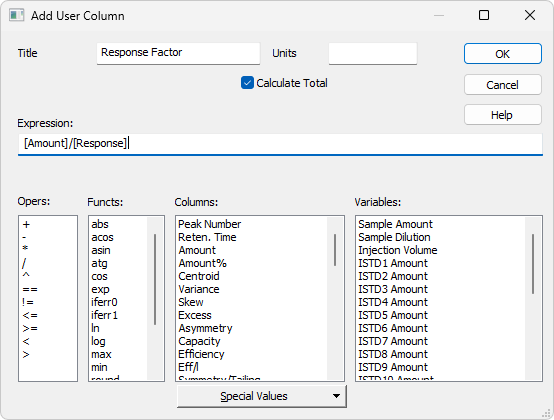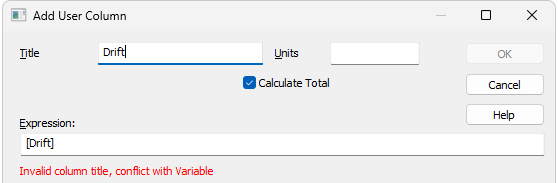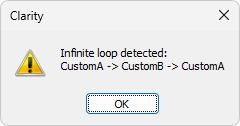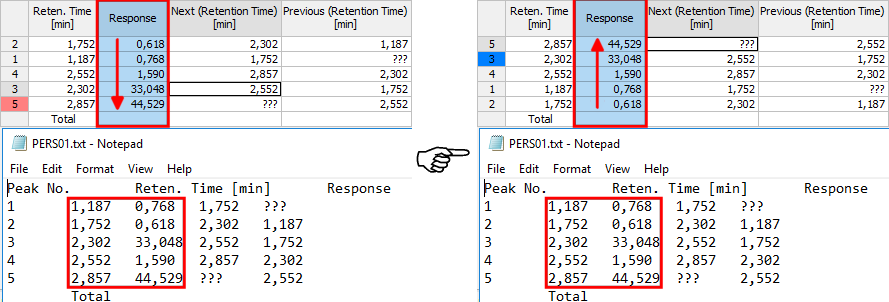Appends a new column with user-defined calculation and opens the Add User Column dialog:Note:

To access the edit of user columns the user must be authorized to edit methods. See the Edit Method checkbox in the User Accounts dialog.

Title

Enter the name of the new column here. Only one line of text is allowed. Title name must be different from default Columns and Variable names, otherwise conflict of names is triggered.Units

Enter the units for the user column.

Calculate Total

Determines whether the Total row in the corresponding User Column should be displayed.

Expression

A calculation formula that can be entered directly or created progressively by double-clicking items in the provided Columns, Opers, Functs, Special Values and Variables fields.

You will be notified if you have composed an expression that causes a recursion, after clicking the OK button.The Details button will appear below the Expression field. Click it to display a message box which will identify the columns that have caused the recursion.Opers

Contains a list of all mathematical operators available. Double-click an operator to insert it in the formula being compiled.

Functs

List of available mathematical functions. Insert a function into an formula by double-clicking the mouse.

List of available functions

Function Explanation
abs - Absolute value
acos - Arcuscosinus (Result is in radians)
asin - Arcussinus (Result is in radians)
atg - Arcustangent (Result is in radians)
cos - Cosinus (Number used for calculation is in radians)
exp - Exponential function
ln - Natural logarithm
log - Logarithm
max - Maximum value of parameter of a function. E.g. MAX (1; 5; 3) returns value 5.
min - Minimum value of parameter of a function. E.g. MIN (1; 5; 3) returns value 1.
round - Rounding off. Number after semicolon specifies number of decimal places. E.g. ROUND (2,8888;2) returns value 2,89.
sin - Sinus (Number used for calculation is in radians)
sqrt - Square root
switch - SWITCH(n; H1; H2; H3;…) returns the value of the nth item Hn
tg - Tangent (Number used for calculation is in radians)

Columns

Lists all available columns as variables. Double-click a column to insert it in the formula (by default as a name of the column in square brackets).

Spec. Values

Calculated values related to the current (selected) column.

List of special values

Value Description
Sum - Sum of all values in the column
Average - Average of the values in the column
Std. Deviation - Standard Deviation of values in the column
Minimum - Minimal value in the column
Maximum - Maximal value in the column
First - Value from the first peak in chromatogram (peak with the lowest retention time)
Last - Value from the last peak in chromatogram (peak with the highest retention time)
Previous - Value from the previous peak in chromatogram (based on peaks' retention order)
Next - Value from the next peak in chromatogram (based on peaks' retention order)
Compound - Opens a table, from which is possible to pickup the name of compound (coined in Calibration or Integration table) and use it as a variable. (e.g. its retention time or amount)

Calculations with applied options First, Last, Previous and Next are based on retention order of peaks in chromatogram. In case the table is resorted (for example according to increasing Response) results for all User Columns will be still calculated according to retention order of peaks in chromatogram. In case the table is exported the Export tool will calculate results for any User Column using any of these options according to retention order of peaks. It means there will be mismatch between order of rows displayed in Clarity and in exported file (or clipboard). Please refer to image below where Results Table is sorted differently but the resulting export file is the same.

It can be read on image below how sorting in (for example) in row Response influences look of the table but does not influence calculated values for User Column. Variously sorted table is exported always the same. Different sorting of table does not cause recalculation of any User Column which uses any of these four options.User Column - Export with Various Sorting of Table

Only peaks displayed in the table are calculated. Due to this, it is possible that the values of these variables may change depending on the setting of the Report in Result Table item from the right side of the Chromatogram - Results tab.

Note:

These functions enable the creation of inter-line calculations, e.g. calculation RRT - relative retention time. For calculating an individual RRT, one can input expression [Reten. Time]/[REFER\$Reten. Time], where REFER will be the name of selected reference compound.

Variables

Lists all available Clarity internal and user's defined variables. Double-click a variable to insert it in the formula.

List of Variable

Value Description
Sample Amount - Defines sample amount.
Sample Dilution - Defines dilution of the sample.
Injection Volume - Defines the volume of injection.
ISTD1-ISTD10 - The amounts of the internal standards for the ISTD method.
Chromatogram Amount - Sum of the amounts across all signals.
Unretained Peak Time - Defines the column dead time.
Column Length - Defines the length of the column.
Noise - Defines the amount of noise in the specifed interval of the chromatogram. The interval is set in the Integration Table.
ASTM Noise - The noise value calculated as an average from values counted for smaller intervals. The interval is set in the Integration Table and divided into same length parts.
6-Sigma Noise - The noise value calculated from the whole interval set in the Integration Table.
Drift - Determines the drift between the beginning and the end point of the specified interval. The interval is set in the Integration Table.
AnalysisUserVar1 - AnalysisUserVar3 - User variables defined for analysis, set in Single Analysis, Sequence or Chromatogram window.
MethodUserVar1 - MethodUserVar3 - User variables defined for method, set in Method Setup window.